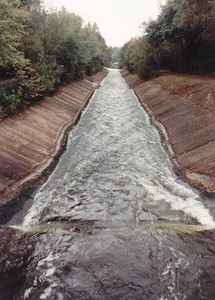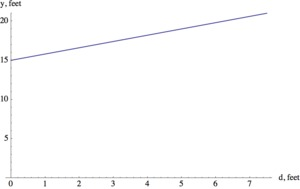# Distance across the channel

Alignments to Content Standards: 8.F.B.4

In science class, Mrs. Winkler’s students are studying the water flow in a channel close to their school (see the photo below found here).A cross section of the channel approximates the shape of an isosceles trapezoid with dimensions noted on the diagrams below: one showing an empty channel and the other the channel with water to the top of its banks.

The students found that there is a linear relationship between the water depth and the distance across the channel at water level. This means that we can write $y$, the distance across the channel at water level (in feet), as a function of the water depth, $d$ (in feet).

1. Use the information in the diagram to find two depth-distance pairs $(d, y)$ that correspond with each other. In other words, find two input-output pairs for the function.
2. Find an equation that describes $y$ as a function of $d$. Draw the graph of the equation.
3. Explain the meaning of the slope and the vertical intercept of the line in the context of the situation. In other words, what does each tell you about the depth of the water and the distance across the channel at water level?
4. What is the distance across the channel at water level when the water is 2 feet deep?

## IM Commentary

This task asks students to find a linear function that models something in the real world. After finding the equation of the linear relationship between the depth of the water and the distance across the channel, students have to verbalize the meaning of the slope and intercept of the line in the context of this situation. Students find this quite hard the first few times they are asked to interpret slopes and intercepts. It is important to give the students repeated opportunities to make connections between mathematical objects and their real world meaning. The slope gives us very specific information about how the depth of the water and the distance across the channel are related and we can describe it in very precise language: "For every one foot increase in water depth, the channel widens by 0.8 feet at water level." It is not enough to say "As the water gets deeper the channel becomes wider".

The task is meant as an instructional task as the complexity of the context (especially the language) would be difficult in an assessment situation. If used for instruction, students should be asked to share their interpretations of the slope and intercept. This could lead to a very productive discussion on precision of language and connections between formulas, graphs and the context of the problem.

There are several ways in which this task could be extended. First, it is possible to prove, using similar triangles, that the relationship between $d$ and $y$ is linear if the cross-section of the channel is an isosceles trapezoid. Another direction entirely would be for an instructor to present the students with the diagram of the channel and ask them to find the amount of water that flows down the channel under different conditions. In this form the task would also illustrate SMP 4 - Model with Mathematics.

## Solutions

Solution: Finding the equation of line

1. We can see from the picture that when there is no water in the channel, i.e. when $d=0$, the distance across the channel is $y=15$ feet. Similarly, when the depth of the water is $d=7.5$ feet, i.e. when the water is at its highest level, the distance across the channel at water level is $y=21$ feet. This gives us two input-output pairs that have to be on the line: $(0,15)$ and $(7.5, 21).$
2. Using the two points on the line, we can compute the slope of the line: $$m=(21-15)/(7.5-0) = 0.8.$$ The vertical intercept is 15, given by the point $(0,15).$ So we have $$y=0.8d+15$$ for the equation of the line. The graph is shown below:3. The slope of the line is $0.8$ horizontal feet per vertical feet. This means that for every one foot increase in water depth, the distance across the channel at water level increases by 0.8 feet. The vertical intercept is $y=15$, this means that when the water depth is 0, i.e. when there is no water in the channel, the distance across the channel is 15 feet.
4. When the water is 2 feet deep the distance across the channel is $0.8\cdot 2+15 = 16.6$ ft.
5. A natural question to ask would be how much water is flowing down the channel during a specific amount of time. The answer depends on the depth of the channel and the velocity of the water. An easier to answer question would be "If the water has the same depth of 2 feet for a 10 foot stretch of the channel, how much water is in that part of the channel"

To compute the amount of water, i.e. the volume, we need to find the blue shaded area in the diagram for $d=2$ and multiply it by 10. We know that the area of a trapezoid with parallel sides of $15$ feet and $16.6$ feet and height $2$ feet is $2\cdot (15+16.6)/2 = 31.6$ square feet. Therefore, the volume of water we are looking for is $31.6\cdot 10 = 316$ cubic feet. Note that we are assuming that the shape of the channel stays the same for the entire 10 foot stretch.

After looking at a few specific questions like this, we can come up with a formula for the water flow down the channel, assuming that the depth of the water and the water velocity stay the same. With these assumptions we can compute how much water is flowing through a particular cross section of the channel at a fixed velocity (e.g. discharge in cubic feet per seconds): $$(\text{area of the cross section of the channel})\cdot (\text{velocity of the water})\cdot (\text{time}).$$

The USGS has a lot of data available for the depth and discharge of many waterways in the US at http://waterdata.usgs.gov/nwis/rt. They also provide a detailed explanation on the methods they use to compute water discharge at http://pubs.usgs.gov/fs/2005/3131/FS2005-3131.pdf

Solution: Alternate for (b)

When there is no water in the channel, the distance across the channel is 15 feet. This tells us that the vertical intercept of the line we are looking for is 15. Furthermore, we can see from the diagram, that when the depth of the water is 7.5 feet, the distance across the channel is 21 feet. This means that the distance increased by 6 feet when the depth increased by 7.5 feet. So for each additional feet of water depth, the distance increased by $\frac{6}{7.5} = 0.8$ feet. Therefore, the equation of the distance as a function of water depth is $$y=15+0.8d.$$